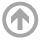Spring 2014 :: Mathematics Department :: USNA
Mathematics Department

# Operator Algebras and Dynamics Seminar

## Spring 2014

All talks are from 3:45-4:45 p.m. in the Seminar room, unless otherwise specified.

• Apr
18
• Cutting and Stacking Constructions in Infinite Ergodic Theory
Prof. Kelly Yancey
University of Maryland

#### View Abstract

In ergodic theory two properties that are very different, though not exclusive, are rigidity and weak mixing. Recall that there are many examples of homeomorphisms of the two-torus that display both of these properties. A natural question to ask is what happens if we consider systems that preserve an infinite measure, like Lebesgue measure on the positive reals. This is part of the set-up in infinite ergodic theory. During this talk we will discuss rigidity verses various types of weak mixing in infinite ergodic theory. We will also construct examples of transformations that have these desired properties. Our examples will be via the method of cutting and stacking. This is joint work with Rachel Bayless.
• Mar
31
• Anti-unitary operators, Hamiltonian operators, and the spectral doubling of normal operators
Prof. Geoffrey Goodson
Towson University

#### View Abstract

Our main result is a spectral pairing theorem for a bounded normal operator \$B\$ which is applied to the study of equations of the form \$KB=B^*K\$ for \$K\$ an antiunitary operator. In particular, using conjugation operators, we generalize the notion of Hamiltonian operator and skew-Hamiltonian operator in a natural way and derive some of their properties.
• Mar
28
• Nonlinear patterns in large sets of rational numbers
Joel Moreira
The Ohio State University

#### View Abstract

An old lemma of Schur states that, given any partition of the positive integer numbers into finitely many pieces, one of the pieces contains a triple of the form {x,y,x+y}. There also exists a triple of the form {x,y,xy} inside a single piece, but it has been an open problem ever since if the same is true for the quadruple {x,y,x+y,xy}. I will describe an approach to this type of problems using dynamical systems and ergodic theory, and I will present recent joint work with V. Bergelson on the analogue of this and similar questions with the set of positive integers replaced with the set of rational numbers.
• Mar
24
• One-parameter flows of von Neumann Algebras
Prof. Alexis Alevras
USNA

#### View Abstract

We will present an expository account of the theory and classification of one parameter semigroups of endomorphisms of von Neumann Algebras, with emphasis on factors of type II and III.
• Feb
11
• Frolik's Theorem and Its Applications (part 2)

#### View Abstract

In this talk, which continues my lecture from 3-Feb-2014, I will apply Frolik's Theorem to prove the following result: If M is a von Neumann algebra, D is a maximal abelian von Neumann subalgebra of M, and v is a partial isometry in M which normalizes D, then there are projections q_0, q_1, q_2, q_3 in D such that (1) q_0 + q_1 + q_2 + q_3 = v^*v, (2) vq_0 is in D, and (3) q_jvq_j = 0 for j = 1, 2, 3. I will indicate various nice consequences of this seemingly technical result.
• Feb
03
• Frolik's Theorem and Its Applications

#### View Abstract

Frolik's Theorem (circa 1968) gives useful information about the structure of homeomorphisms between clopen subsets of an extremally disconnected Hausdorff space. Specifically, it says that the set of fixed points of such a map is clopen, and that the non-fixed points can be partitioned into three clopen sets, each of which is disjoint from its image under the homeomorphism. In this expository talk, we prove Frolik's Theorem and explain some of its applications to von Neumann algebra theory (specifically to the structure of partial isometries in a von Neumann algebra which normalize a maximal abelian von Neumann subalgebra).
• Jan
24
• Topologically Mixing Tiling of R^2 Generated by a Generalized Substitution
Prof. Tyler White
Northern Virginia Community College
• Jan
10
• On homeomorphisms that are weakly mixing and rigid
Prof. Kelly Yancey
University of Maryland

#### View Abstract

During this talk we will discuss what it means for a dynamical system to be rigid and weakly mixing. These are very different properties of a dynamical system, though not exclusive. We will discuss these concepts in ergodic theory and topological dynamics. We will also give partial results toward characterizing (uniform) rigidity sequences for weakly mixing systems.

#### ARCHIVES